Back to Contents

# Making Lists

A list is a collection of elements. Here is a list of three integers:

`[1; 2; 3]`

We write a list between square brackets `[` and `]`, separating the elements with semicolons. The list above has type int list, because it is a list of integers. All elements of the list must have the same type. The elements in the list are ordered (in other words, `[1; 2; 3]` and `[2; 3; 1]` are not the same list).

The first element is called the head, and the rest are collectively called the tail. In our example, the head is the integer `1` and the tail is the list `[2; 3]`. So you can see that the tail has the same type as the whole list. Here is a list with no elements (called “the empty list” or sometimes “nil”):

`[]`

It has neither a head nor a tail. Here is a list with just a single element:

``

Its head is the integer `5` and its tail is the empty list `[]`. So every non-empty list has both a head and a tail. Lists may contain elements of any type: integers, booleans, functions, even other lists. For example, here is a list containing elements of type bool:

`[false; true; false]`  :  bool list

OCaml defines two operators for lists. The `::` operator (pronounced “cons”) is used to add a single element to the front of an existing list: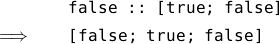The cons operation is completed in a constant amount of time, regardless of the length of the list. The `@` operator (pronounced “append”) is used to combine two lists together: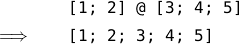This takes time proportional to the length of the list on the left hand side of the `@` operator (that is, a list of length 100 will take roughly twice as long as one of length 50). We will see why soon.

Now, how do we write functions using lists? We can use pattern matching as usual, with some new types of pattern. For example, here’s a function which tells us if a list is empty: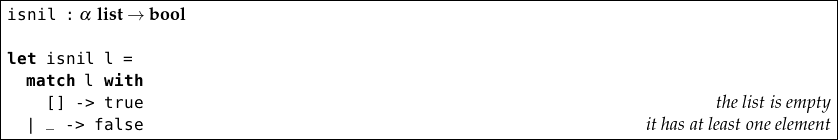The argument has type α list (which OCaml prints on the screen as `’a list`) because this function does not inspect the individual elements of the list, it just checks if the list is empty. And so, this function can operate over any type of list. The greek letters α, β, γ etc. stand for any type. If two types are represented by the same greek letter they must have the same type. If they are not, they may have the same type, but do not have to. Functions like this are known as polymorphic. We can also use `::` in our patterns, this time using it to deconstruct rather than construct the list: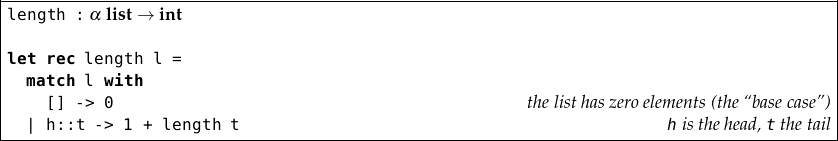Here is how the evaluation proceeds: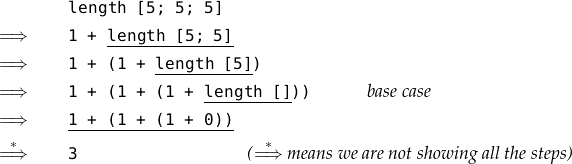This works by recursion over the list, then addition of all the resultant `1`s. It takes time proportional to the length of the list. Can you see why? It also takes space proportional to the length of the list, because of the intermediate expression `1 + (1 + (1 + …` which is built up before any of the `+` operations are evaluated – this expression must be stored somewhere whilst it is being processed. Since `h` is not used in the expression `1 + length t`, this function is also polymorphic. Indeed we can replace `h` in the pattern with `_` since there is no use giving a name to something we are not going to refer to: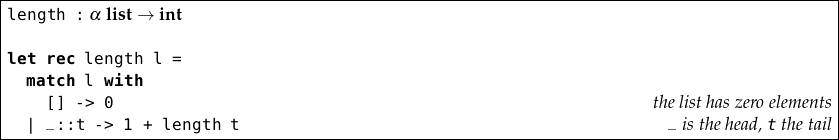A very similar function can be used to add a list of integers: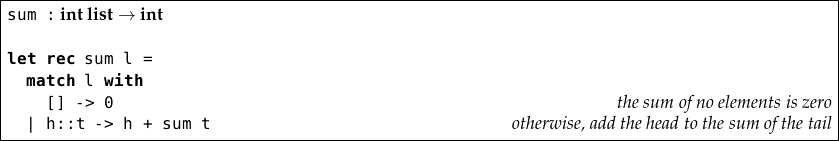However, since we are actually using the individual list elements (by adding them up), this function is not polymorphic – it operates over lists of type int list only. If we accidentally miss out a case, OCaml will alert us, and give an example pattern which is not matched: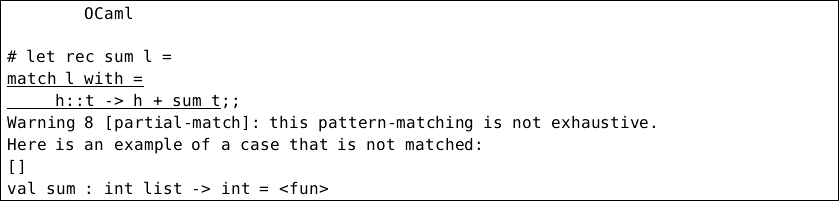There is a way to deal with the excessive space usage from the building up of a large intermediate expression `1 + 1 + 1 + …` in our `length` function, at the cost of readability. We can “accumulate” the `1s` as we go along in an extra argument. At each recursive step, the accumulating argument is increased by one. When we have finished, the total is returned: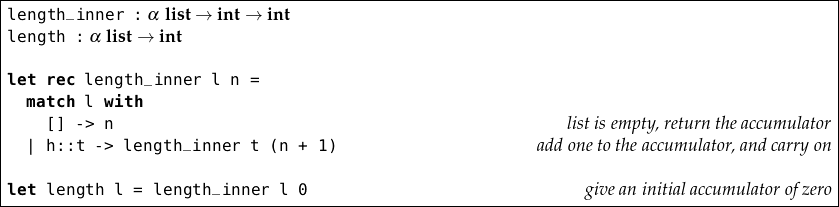We wrapped it up in another function to make sure we do not call it with a bad initial value for the accumulating argument. Here is an example evaluation: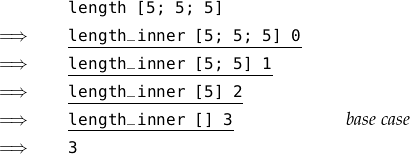Now, the space taken by the calculation does not relate in any way to the length of the list argument. Recursive functions which do not build up a growing intermediate expression are known as tail recursive. Functions can, of course, return lists too. Here is a function to return the list consisting of the first, third, fifth and so on elements in a list: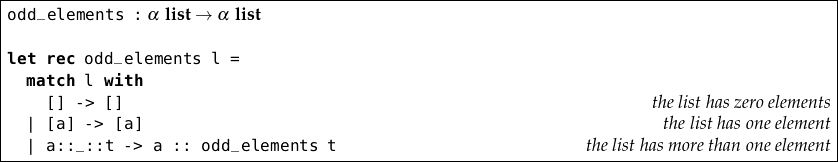Consider the evaluation of `odd_elements [2; 4; 2; 4; 2]`: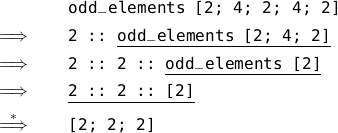You might notice that the first two cases in the pattern match return exactly their argument. By reversing the order, we can reduce this function to just two cases: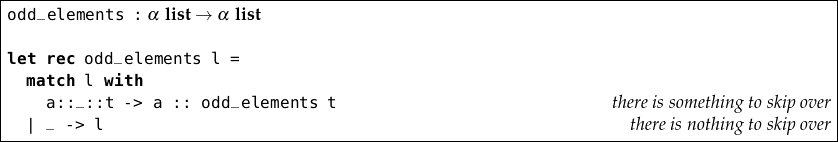We have seen how to use the `@` (append) operator to concatenate two lists: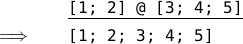How might we implement list append ourselves, if it was not provided? Consider a function `append a b`. If the list `a` is the empty list, the answer is simply `b`. But what if `a` is not empty? Then it has a head `h` and a tail `t`. So we can start our result list with the head, and the rest of the result is just `append t b`.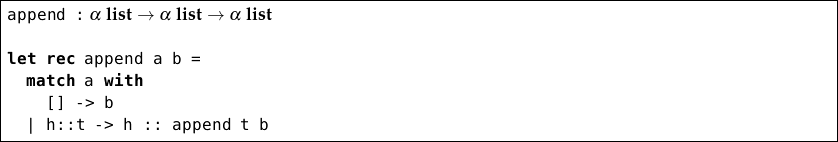Consider the evaluation of `append [1; 2; 3] [4; 5; 6]`: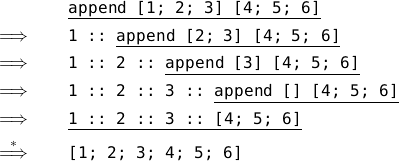This takes time proportional to the length of the first list – the second list need not be processed at all. What about reversing a list? For example, we want `rev [1; 2; 3; 4]` to evaluate to `[4; 3; 2; 1]`. One simple way is to reverse the tail of the list, and append the list just containing the head to the end of it: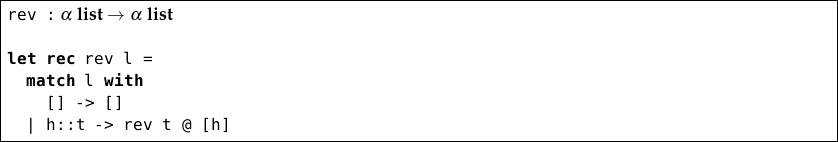Here’s how the evaluation proceeds: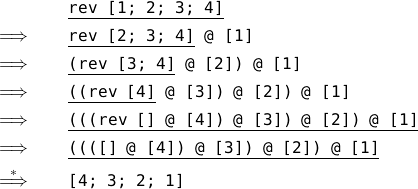This is a simple definition, but not very efficient – can you see why?

Two more useful functions for processing lists are `take` and `drop` which, given a number and a list, either take or drop that many elements from the list: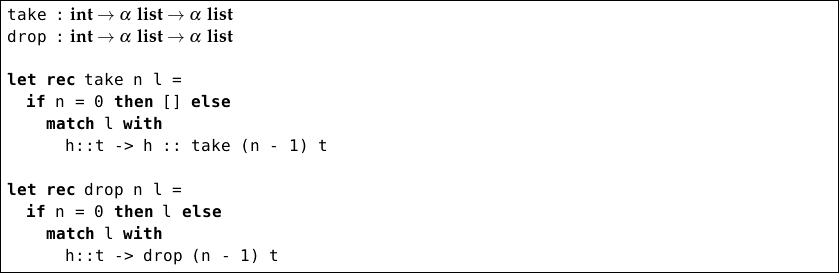For example, here’s the evaluation for `take 2 [2; 4; 6; 8; 10]`: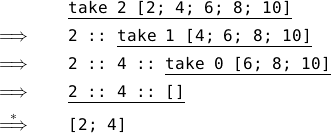And for `drop 2 [2; 4; 6; 8; 10]`: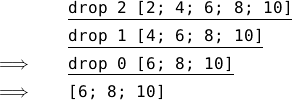Note that these functions contain incomplete pattern matches – OCaml tells us so when we type them in. The function fails if the arguments are not sensible – that is, when we are asked to take or drop more elements than are in the argument list. Later on, we will see how to deal with that problem. Note also that for any sensible value of `n`, including zero, `take n l` and `drop n l` split the list into two parts with no gap. So `drop` and `take` often appear in pairs.

Lists can contain anything, so long as all elements are of the same type. So, of course, a list can contain lists. Here’s a list of lists of integers:

`[; [2; 3]; [4; 5; 6]]`  :  (int list) list

Each element of this list is of type int list (we can also omit the parentheses and write int list list). Within values of this type, it is important to distinguish the list of lists containing no elements

`[] :` α list list

from the list of lists containing one element which is the empty list

`[[]] :` α list list

## Questions

1. Write a function `evens` which does the opposite to `odds`, returning the even numbered elements in a list. For example, `evens [2; 4; 2; 4; 2]` should return `[4; 4]`. What is the type of your function?

2. Write a function `count_true` which counts the number of `true` elements in a list. For example, `count_true [true; false; true]` should return `2`. What is the type of your function? Can you write a tail recursive version?

3. Write a function which, given a list, builds a palindrome from it. A palindrome is a list which equals its own reverse. You can assume the existence of `rev` and `@`. Write another function which determines if a list is a palindrome.

4. Write a function `drop_last` which returns all but the last element of a list. If the list is empty, it should return the empty list. So, for example, `drop_last [1; 2; 4; 8]` should return `[1; 2; 4]`. What about a tail recursive version?

5. Write a function `member` of type α α list bool which returns `true` if an element exists in a list, or `false` if not. For example, `member 2 [1; 2; 3]` should evaluate to `true`, but `member 3 [1; 2]` should evaluate to `false`.

6. Use your `member` function to write a function `make_set` which, given a list, returns a list which contains all the elements of the original list, but has no duplicate elements. For example, `make_set [1; 2; 3; 3; 1]` might return `[2; 3; 1]`. What is the type of your function?

7. Can you explain why the `rev` function we defined is inefficient? How does the time it takes to run relate to the size of its argument? Can you write a more efficient version using an accumulating argument? What is its efficiency in terms of time taken and space used?